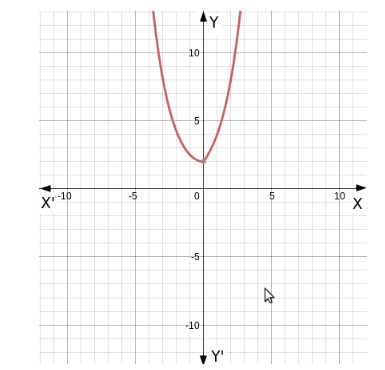# The function f : R → R defined by

Question:

The function $f: R \rightarrow R$ defined by $f(x)=2^{x}+2^{|x|}$ is

(a) one-one and onto
(b) many-one and onto
(c) one-one and into
(d) many-one and into

Solution:

(d) many-one and into

Graph for the given function is as follows.A line parallel to $X$ axis is cutting the graph at two different values.

Therefore, for two different values of x we are getting the same value of y.
That means it is many one function.

From the given graph we can see that the range is $[2, \infty)$

and R is the co-domain of the given function.
Hence, Co-domain">Range
Therefore, the given function is into.